Next: Ohm's Law Up: Electric Current Previous: Electric Current

## Electric Circuits

A battery is a device possessing a positive and a negative terminal. Some process, usually a chemical reaction, takes place inside the battery which causes positive charge to migrate towards the positive terminal, and vice versa. This process continues until the electric field set up between the two terminals is sufficiently strong to inhibit any further charge migration.

An electric circuit is a conducting path, external to the battery, which allows charge to flow from one terminal to the other. A simple circuit might consist of a single strand of metal wire linking the positive and negative terminals. A more realistic circuit possesses multiple branch points, so that charge can take many different paths between the two terminals.

Suppose that a (positive) chargeis driven around the external circuit, from the positive to the negative terminal, by the electric field set up between the terminals. The work done on the charge by this field during its journey is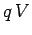, whereis the difference in electric potential between the positive and negative terminals. We usually refer toas the voltage of the battery: e.g., when we talk of a 6 volt battery, what we actually mean is that the potential difference between its two terminals is 6 V. Note, from Sect. 5, that the electrical workdone on the charge is completely independent of the route it takes between the terminals. In other words, although there are, in general, many different paths through the external circuit which the charge could take in order to get from the positive to the negative terminal of the battery, the electrical energy which the charge acquires in making this journey is always the same. Since, when analyzing electrical circuits, we are primarily interested in energy (i.e., in the transformation of the chemical energy of the battery into heat energy in some electric heating element, or mechanical energy in some electric motor, etc.), it follows that the property of a battery which primarily concerns us is its voltage. Hence, we do not have to map out the electric field generated by a battery in order to calculate how much energy this field gives to a chargewhich goes around some external circuit connected to it. All we need to know is the potential differencebetween the two terminals of the battery. This is obviously an enormous simplification.

This section is only concerned with steady-state electric circuits powered by batteries of constant voltage. Thus, the rate at which electric charge flows out of the positive terminal of the battery into the external circuit must match the rate at which charge flows from the circuit into the negative terminal of the battery, otherwise charge would build up in either the battery or the circuit, which would not correspond to a steady-state situation. The rate at which charge flows out of the positive terminal is termed the electric current flowing out of the battery. Likewise, the rate at which charge flows into the negative terminal is termed the current flowing into the battery. Of course, these two currents must be the same in a steady-state. Electric current is measured in units of amperes (A), which are equivalent to coulombs per second: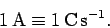(124)

We can define the electric currentflowing at any particular point in the external circuit as follows. If an amount of charge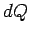flows past this point in an infinitesimal time interval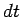then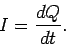(125)

By convention, the direction of the current is taken to be the direction positive charges would have to move in order to account for the flow of charge. In a steady-state, the current at all points in the external circuit must remain constant in time. We call this type of circuit a direct current (DC) circuit because the current always flows in the same direction. There is a second type of circuit, called an alternating current (AC) circuit, in which the current periodically switches direction.

Consider a simple circuit in which a steady currentflows around a single conducting wire connecting the positive and negative terminals of a battery of voltage. Let us suppose that the current is carried by positive charges flowing around the external circuit from the positive to the negative terminal. In reality, the current is carried by negative charges (i.e., by electrons) flowing in the opposite direction, but for most purposes we can safely ignore this rather inconvenient fact. Every chargewhich flows around the external circuit experiences a potential drop. In order to flow around the circuit again, the charge must be raised to the potential of the positive terminal of the battery. This process occurs inside the battery, as the charge migrates from the negative to the positive terminal. The energyrequired to move the charge between the two terminals is derived from the energy released by the chemical reactions taking place inside the battery.

The simple circuit described above is somewhat analogous to a small ski resort. The charges flowing around the external circuit are like people skiing down the ski-slope. The charges flow down a gradient of electric potential just as the people ski down a gradient of gravitational potential. Note that the good skiers who ski directly down the slope acquire exactly the same gravitational energy as the poor skiers who ski from side to side. In both cases, the total acquired energy depends only on the difference in height between the top and bottom of the slope. Likewise, charges flowing around an external circuit acquire the same electrical energy no matter what route they take, because the acquired energy only depends on the potential difference between the two terminals of the battery. Once the people in our ski resort reach the bottom of the slope, they must be lifted to the top in a ski-lift before they can ski down it again. Thus, the ski-lift in our resort plays an analogous role to the battery in our circuit. Of course, the ski-lift must expend non-gravitational energy in order to lift skiers to the top of the slope, in just the same manner as the battery must expend non-electrical energy to move charges up a potential gradient. If the ski-lift runs out of energy then the circulation of skiers in the resort rapidly stops. Likewise, if the battery runs out of energy (i.e., if the battery runs down'') then the current in the external circuit stops flowing.Next: Ohm's Law Up: Electric Current Previous: Electric Current
Richard Fitzpatrick 2007-07-14